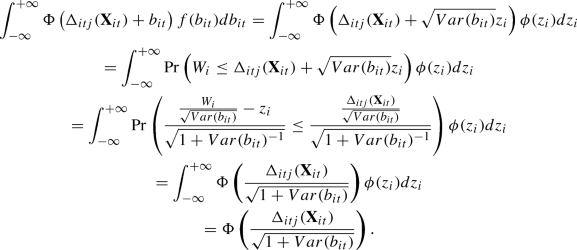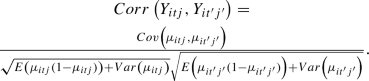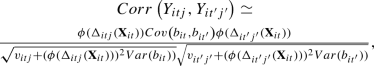# A marginalized multilevel model for bivariate longitudinal binary data

A Publisher Correction to this article was published on 12 September 2019

## Abstract

This study considers analysis of bivariate longitudinal binary data. We propose a model based on marginalized multilevel model framework. The proposed model consists of two levels such that the first level associates the marginal mean of responses with covariates through a logistic regression model and the second level includes subject/time specific random intercepts within a probit regression model. The covariance matrix of multiple correlated time-specific random intercepts for each subject is assumed to represent the within-subject association. The subject-specific random effects covariance matrix is further decomposed into its dependence and variance components through modified Cholesky decomposition method and then the unconstrained version of resulting parameters are modelled in terms of covariates with low-dimensional regression parameters. This provides better explanations related to dependence and variance parameters and a reduction in the number of parameters to be estimated in random effects covariance matrix to avoid possible identifiability problems. Marginal correlations between responses of subjects and within the responses of a subject are derived through a Taylor series-based approximation. Data cloning computational algorithm is used to compute the maximum likelihood estimates and standard errors of the parameters in the proposed model. The validity of the proposed model is assessed through a Monte Carlo simulation study, and results are observed to be at acceptable level. Lastly, the proposed model is illustrated through Mother’s Stress and Children’s Morbidity study data, where both population-averaged and subject-specific interpretations are drawn through Emprical Bayes estimation of random effects.

This is a preview of subscription content, access via your institution.

## Change history

• ### 12 September 2019

Unfortunately, due to a technical error, the articles published in issues 60:2 and 60:3 received incorrect pagination. Please find here the corrected Tables of Contents. We apologize to the authors of the articles and the readers.

## References

1. Asar O, Ilk O (2013) pnmtrem: probit-normal marginalized transition random effects models. R package version 1.3. http://cran.r-project.org/web/packages/pnmtrem

2. Asar O, Ilk O (2014) mmm: multivariate marginal models. R package version 1.4. http://cran.r-project.org/web/packages/mmm

3. Asar O, Ilk O (2016) First-order marginalised transition random effects models with probit link function. J App Stat 43:925–942

4. Ballings M, Poel D Van den (2013) AUC: threshold independent performance measures for probabilistic classifiers. R package version 0.3.0. http://cran.r-project.org/web/packages/AUC/index.html

5. Capanu M, Gonen M, Colin B (2013) An assessment of estimation methods for generalized linear mixed models with binary outcomes. Stat Med 32:4550–4566

6. Carey V (2012) gee: generalized estimation equation solver. R package version 4.13-18. http://CRAN.R-project.org/package=gee

7. Daniels M, Zhao Y (2003) Modelling the random effects covariance matrix in longitudinal data. Stat Med 22:1631–1647

8. Diggle P, Heagerty P, Liang K, Zeger S (2002) Analysis of longitudinal data. Oxford University Press, New York

9. Efendi A, Molenberghs G, Njagi E, Dendale P (2013) A joint model for longitudinal and time-to-event outcomes with direct marginal interpretation. Biom J 55:572–588

10. Goldstein H, Rasbash J (1996) Improved approximations for multilevel models with binary responses. J R Stat Soc Ser B 159:505–513

11. Griswold M (2005) Complex distributions, hmmmm. Hierarchical mixtures of marginalized multilevel models. Ph.D Thesis. Department of Biostatistics, Johns Hopkins University, USA

12. Griswold M, Swihart B, Caffo B, Zeger S (2013) Practical marginalized multilevel models. Statistics 2:129–142

13. Guo C, Yang H, Lv J (2016) Two step estimations for a single-index varying-coeffcient model with longitudinal data. Stat Pap 17(1):1–27

14. Heagerty P (1999) Marginally specified logistic-normal models for longitudinal binary data. Biometrics 55:688–698

15. Heagerty P (2002) Marginalized transition models and likelihood inference for longitudinal categorical data. Biometrics 58:342–351

16. Heagerty P, Zeger S (2000) Marginalized multilevel models and likelihood inference (with discussion). Stat Sci 15:1–26

17. Iddi S, Molenberghs G (2012) A joint marginalized multilevel model for longitudinal outcomes. J App Stat 39:2413–2430

18. Ilk O, Daniels M (2007) Marginalized transition random effects models for multivariate longitudinal binary data. Can J Stat 35:105–123

19. Inan G (2014) Marginalized multilevel model for bivariate longitudinal binary data. Ph.D thesis. Middle East Technical University, Turkey

20. Kim Y, Choi Y, Emery S (2013) Logistic regression with multiple random effects: a simulation study of estimation methods and statistical packages. Am Stat 67:171–182

21. Lee K (2013) Bayesian modeling of random effects covariance matrix for generalized linear mixed models. CSAM 20:235–240

22. Lee K, Joo Y, Yoo J, Lee J (2009) Marginalized random effects models for multivariate longitudinal binary data. Stat Med 28:1284–1300

23. Lee K, Lee J, Hagan J, Yoo J (2012) Modeling the random effects covariance matrix for generalized linear mixed models. Comput Stat Data Anal 56:1545–1551

24. Lee K, Daniels M, Joo Y (2013) Flexible marginalized models for bivariate longitudinal ordinal data. Biostatistics 14:462–476

25. Lele S, Dennis B, Lutscher F (2007) Data cloning: easy maximum likelihood estimation for complex ecological models using Bayesian Markov chain Monte Carlo methods. Ecol Lett 10:551–563

26. Lele S, Nadeem K, Schmuland B (2010) Estimability and likelihood inference for generalized linear mixed models using data cloning. J Am Stat Assoc 105:1617–1625

27. Li E, Pourahmadi M (2013) An alternative REML estimation of covariance matrices in linear mixed models. Stat Probab Lett 83:1071–1077

28. Njagi E, Molenberghs G, Rizopolous D, Verbeke G, Kenward M, Dendale P, Willekens K (2013) A flexible joint modeling framework for longitudinal and time-to-event data with overdispersion. Stat Methods Med Res 1:1–16

29. Pan J, Mackenzie G (2007) Modelling conditional covariance in the linear mixed model. Stat Model 7:49–71

30. Ponciano J, Taper M, Dennis B, Lele S (2009) Hierarchical models in ecology: confidence intervals, hypothesis testing, and model selection using data cloning. Ecology 90:356–362

31. Pourahmadi M (1999) Joint mean-covariance models with applications to longitudinal data: unconstrained parameterization. Biometrika 86:677–690

32. Pourahmadi M (2011) Covariance estimation: the GLM and regularization perspectives. Stat Sci 26:369–387

33. Rizopoulos D, Lesaffre E (2014) Joint modeling techniques. Stat Methods Med Res 23:3–10

34. Rudary M (2009) On predictive linear gaussian models. Ph.D Thesis. University of Michigan, USA

35. Sólymos P (2010) dclone: data cloning in R. R J 2:29–37

36. Torabi M (2013) Likelihood inference in generalized linear mixed measurement error models. Comput Stat Data Anal 57:549–557

37. Vangeneugden T, Molenberghs G, Laenen A, Geys H, Beunckens C, Sotto C (2010) Marginal correlation in longitudinal binary data based on generalized linear mixed models. Comun Stat Theory Methods 39:3540–3557

38. Vangeneugden T, Molenberghs G, Verbeke G, Demetrio C (2011) Marginal correlation from an extended random-effects model for repeated and overdispersed counts. J App Stat 38:215–232

39. Withanage N, de Leon A, Rudnisky C (2015) Joint estimation of multiple disease-specific sensitivities and specificities via crossed random effects models for correlated reader-based diagnostic data: application of data cloning. Stat Med 34:3916–3928

## Acknowledgements

Authors would like to thank to Assoc. Prof. Dr. Alexander de Leon for suggesting the use of data cloning computational algorithm in this study. Gul Inan would like to thank to The Scientific and Technological Research Council of Turkey (TUBITAK) for funding her studies through 2211-A and 2219 Fellowship Programmes. Authors would also like to thank the editor and two anonymous reviewers for their constructive comments which improved the quality of the paper.

## Author information

Authors

### Corresponding author

Correspondence to Gul Inan.

## Appendix

### Detailed calculations of $$\Delta _{{itj}}$$

In probability theory, it is known that any conditional expectation can be written in terms of marginal expectation. This implies that the integration of conditional probability $$Pr(Y_{itj}=1|\mathbf {X}_{it},b_{it})$$ over the distribution of random effect results in marginal probability $$Pr(Y_{itj}=1|\mathbf {X}_{it})$$ for random effects models for longitudinal binary data, as given follows:

\begin{aligned} \begin{aligned} E(Y_{itj}|\mathbf {X}_{it})&=\int E(Y_{itj}|\mathbf {X}_{it},b_{it} )f(b_{it})db_{it} \\ \Rightarrow Pr(Y_{itj}=1|\mathbf {X}_{it})&= \int Pr(Y_{it}=1|\mathbf {X}_{it},b_{it})f(b_{it})db_{it}, \end{aligned} \end{aligned}
(14)

where $$f(b_{it})$$ is a univariate normal distribution with mean 0 and variance $$Var(b_{it})$$. Substituting marginal and conditional probabilities given in Eq. 1, which are the first and second levels of the proposed model, into Eq. 14 gives the following expression:

\begin{aligned} \frac{exp(\mathbf {X}_{it}\varvec{\beta }_{j})}{1+exp(\mathbf {X}_{it}\varvec{\beta }_{j})}&=\int ^{+\infty }_{-\infty } \Phi (\Delta _{itj}(\mathbf {X}_{it})+ b_{it})f(b_{it}) db_{it}. \end{aligned}
(15)

Since $$b_{it}\sim N(0,Var(b_{it}))$$, it is possible to write $$b_{it}=\sqrt{Var(b_{it})}z_{i}$$, where $$z_{i}\sim N(0,1)$$. Following Griswold (2005), define a $$W_{i}\sim N(0,1)$$, where $$W_{i}\perp z_{i}$$ and the symbol $$\perp$$ denotes independence. Then it is easy to show that

\begin{aligned} \begin{array}{ll} \frac{W_{i}}{\sqrt{Var(b_{it})}}-z_{i}&\sim N(0,1 + Var(b_{it})^{-1}). \end{array} \end{aligned}
(16)

The right-hand side of Eq. 15 can be rewritten as follows:Then Eq. 15 can be rewritten as follows:

\begin{aligned} \frac{exp(\mathbf {X}_{it}\varvec{\beta }_{j})}{1+exp(\mathbf {X}_{it}\varvec{\beta }{j})}&=\Phi \left( \frac{\Delta _{itj}(\mathbf {X}_{it})}{\sqrt{1+ Var(b_{it})}}\right) . \end{aligned}
(17)

Solving Eq. 17 for $$\Delta _{itj}$$ provides a closed-form solution for it such that

\begin{aligned} \Delta _{itj}= \Phi ^{-1} \left( \frac{exp(\mathbf {X}_{it}\varvec{\beta }_{j})}{1+exp(\mathbf {X}_{it}\varvec{\beta }_{j})}\right) \sqrt{1+ Var(b_{it})}, \end{aligned}
(18)

$$\forall$$i, t and j, where $$\Delta _{itj}$$ is an explicit function of both marginal regression parameter (i.e., $$\varvec{\beta }_{j}$$) and variance of random effect (i.e., Var($$b_{it}$$)).

### Taylor series-based derivation of marginal correlation function

The formula for the marginal correlation between $$Y_{itj}$$ and $$Y_{it^{\prime }j^{\prime }}$$, $$Corr\left( Y_{itj}, Y_{it^{\prime }j^{\prime }}\right)$$ is given as follows:

\begin{aligned} \begin{array}{ll} Corr\left( Y_{itj}, Y_{it^{\prime }j^{\prime }}\right)&=\frac{Cov\left( Y_{itj}, Y_{it^{\prime }j^{\prime }}\right) }{\sqrt{Var(Y_{itj})} \sqrt{Var(Y_{it^{\prime }j^{\prime }})} } \quad \forall i, t\,\text {and}\,j, \end{array} \end{aligned}
(19)

where $$Cov\left( Y_{itj}, Y_{it^{\prime }j^{\prime }}\right)$$ is covariance between $$Y_{itj}$$ and $$Y_{it^{\prime }j^{\prime }}$$ and $$Var(Y_{itj})$$ is variance of $$Y_{itj}$$.

For any model including random effects, the formulation for $$Corr\left( Y_{itj}, Y_{it^{\prime }j^{\prime }}\right)$$ in Eq. 19 relies on conditional expectation, variance, and covariance formulas. The marginal covariance $$Cov\left( Y_{itj}, Y_{it^{\prime }j^{\prime }}\right)$$ can be formulated in terms of conditional expectation and covariance as follows (Rudary 2009):

\begin{aligned} Cov\left( Y_{itj},Y_{it^{\prime }j^{\prime }}\right) \!\!=\!E\left( Cov\left( Y_{itj},Y_{it^{\prime }j^{\prime }}|b_{it},b_{it^{\prime }}\right) \right) \!+\!Cov\left( E\left( Y_{itj}|b_{it}\right) , E\left( Y_{it^{\prime }j^{\prime }}|b_{it^{\prime }}\right) \right) , \end{aligned}
(20)

where $$E\left( Cov\left( Y_{itj},Y_{it^{\prime }j^{\prime }}|b_{it},b_{it^{\prime }}\right) \right) =E\left( E[(Y_{itj}-\mu _{itj})\times (Y_{it^{\prime }j^{\prime }}-\mu _{it^{\prime }j^{\prime }})]|b_{it}, b_{it^{\prime }}\right)$$$$=E\left( E\left( Y_{itj}-\mu _{itj}\right) \times E\left( Y_{it^{\prime }j^{\prime }}-\mu _{it^{\prime }j^{\prime }}\right) \right) =0$$, with $$E\left( Y_{itj}|b_{it}\right) =\mu _{itj}$$, since $$Y_{itj}|b_{it}$$ and $$Y_{it^{\prime }j^{\prime }}|b_{it^{\prime }}$$ are independent of each other, which is also known as the conditional independence assumption, and $$Cov\left( E\left( Y_{itj} |b_{it} \right) , E\left( Y_{it^{\prime }j^{\prime }} |b_{it^{\prime }} \right) \right) = Cov\left( \mu _{itj},\mu _{it^{\prime }j^{\prime }} \right)$$. Then $$Cov\left( Y_{itj}, Y_{it^{\prime }j^{\prime }}\right)$$ in Eq. 20 can be rewritten as follows:

\begin{aligned} \begin{array}{ll} Cov\left( Y_{itj}, Y_{it^{\prime }j^{\prime }}\right)&=Cov\left( \mu _{itj},\mu _{it^{\prime }j^{\prime }} \right) . \end{array} \end{aligned}
(21)

The marginal variance $$Var(Y_{itj})$$ can be formulated in terms of conditional expectation and variance as follows:

\begin{aligned} \begin{array}{ll} Var(Y_{itj})&=E\left( Var\left( Y_{itj}|b_{it}\right) \right) + Var\left( E\left( Y_{itj}|b_{it}\right) \right) , \end{array} \end{aligned}
(22)

where $$E\left( Var\left( Y_{itj}|b_{it}\right) \right) =E\left( \mu _{itj}(1-\mu _{itj}) \right)$$ and $$Var\left( E\left( Y_{itj}|b_{it}\right) \right) =Var\left( \mu _{itj}\right)$$. Then $$Var(Y_{itj})$$ in Eq. 22 can be rewritten as follows:

\begin{aligned} \begin{array}{ll} Var(Y_{itj})&=E\left( \mu _{itj}(1-\mu _{itj})\right) + Var\left( \mu _{itj}\right) . \end{array} \end{aligned}
(23)

Then $$Corr\left( Y_{itj},Y_{it^{\prime }j^{\prime }}\right)$$ in Eq. 19 can be rewritten based on Eqs. 21 and 23 as follows:(24)

#### Taylor series-based approximations for $$E(\mu _{itj}(1-\mu _{itj})), Var(\mu _{itj})$$ and $$Cov(\mu _{itj}, \mu _{it^{\prime }j^{\prime }})$$

Equation 24 requires a closed-form solution for $$E(\mu _{itj}(1-\mu _{itj}))$$, $$Var(\mu _{itj})$$ and $$Cov(\mu _{itj}, \mu _{it^{\prime }j^{\prime }})$$. However, unless the link function in $$\mu _{itj}$$ is an identity function, a closed-form solution for these expressions cannot be obtained (e.g., for binary responses). In this sense, following Goldstein and Rasbash (1996), Vangeneugden et al. (2010), and Vangeneugden et al. (2011), these expressions can be approximated by a first-order Taylor series expansion around $$b_{it}=0$$.

First-order Taylor series expansions of $$E(\mu _{itj}(1-\mu _{itj}))$$ and $$Var(\mu _{itj})$$ around $$b_{it}=0$$ give the following approximations, respectively:

\begin{aligned} E(\mu _{itj}(1-\mu _{itj}))\simeq (\mu _{itj}(1-\mu _{itj})|_{b_{it}=0}) \quad \text{ and } \end{aligned}
(25)
\begin{aligned} Var(\mu _{itj})&\simeq \left( \frac{\partial \mu _{itj}}{\partial b_{it}}|_{b_{it}=0}\right) ^2 Var(b_{it}). \end{aligned}
(26)

In a similar fashion, a first-order Taylor series expansion for $$Cov(\mu _{itj}, \mu _{it^{\prime }j^{\prime }})$$ around $$b_{it}=0$$ and $$b_{it^{\prime }}=0$$ gives the following approximation:

\begin{aligned} \begin{array}{ll} Cov\left( Y_{itj},Y_{it^{\prime }j^{\prime }}\right)&\simeq \left( \frac{\partial \mu _{itj}}{\partial b_{it}}|_{b_{it}=0}\right) Cov\left( b_{it},b_{it^{\prime }}\right) \left( \frac{\partial \mu _{it^{\prime }j^{\prime }}}{\partial b_{it^{\prime }}}|_{b_{it^{\prime }}=0}\right) . \end{array} \end{aligned}
(27)

For the proposed model in Eq. 1, $$\mu _{itj}= Pr(Y_{itj}=1|\mathbf {X}_{it},b_{it}) = \Phi (\Delta _{itj}(\mathbf {X}_{it})+ b_{it})$$. Then Eqs. 25, 26 and 27 can be rearranged, respectively, as follows:

\begin{aligned} \begin{array}{ll} E(\mu _{itj}(1-\mu _{itj}))&{}\simeq (\mu _{itj}(1-\mu _{itj})|_{b_{it}=0}) \\ &{}= \Phi (\Delta _{itj}(\mathbf {X}_{it})) (1-\Phi (\Delta _{itj}(\mathbf {X}_{it}))), \end{array} \end{aligned}
(28)
\begin{aligned} \begin{array}{ll} Var(\mu _{itj})&{}\simeq \left( \frac{\partial \mu _{itj}}{\partial b_{it}}|_{b_{it}=0}\right) ^2 Var(b_{it})\\ &{}=(\phi (\Delta _{itj}(\mathbf {X}_{it})))^2 Var(b_{it}), \end{array} \end{aligned}
(29)

and

\begin{aligned} \begin{array}{ll} Cov\left( Y_{itj},Y_{it^{\prime }j^{\prime }}\right) &{}\simeq \left( \frac{\partial \mu _{itj}}{\partial b_{it}}|_{b_{it}=0}\right) Cov\left( b_{it},b_{it^{\prime }}\right) \left( \frac{\partial \mu _{it^{\prime }j^{\prime }}}{\partial b_{it^{\prime }}}|_{b_{it^{\prime }}=0}\right) \\ &{}=\phi (\Delta _{itj}(\mathbf {X}_{it}))Cov\left( b_{it},b_{it^{\prime }}\right) \phi (\Delta _{it^{\prime }j^{\prime }}(\mathbf {X}_{it})), \end{array} \end{aligned}
(30)

where $$Var(b_{it})$$ and $$Cov(b_{it},b_{it^{\prime }})$$ are the corresponding variance and covariance components in $$\Sigma _{i}$$.

For the proposed model in Eq. 1, $$Corr(Y_{itj},Y_{it^{\prime }j^{\prime }})$$ can be expressed through Eqs. 2830 as follows:(31)

$$\forall$$i, t and j, where $$v_{itj}=\Phi (\Delta _{itj}(\mathbf {X}_{it})) (1-\Phi (\Delta _{itj}(\mathbf {X}_{it})))$$, $$v_{itj^{\prime }}=\Phi (\Delta _{itj^{\prime }}(\mathbf {X}_{it})) (1-\Phi (\Delta _{itj^{\prime }}(\mathbf {X}_{it})))$$, $$\phi (.)$$ is the probability density function of standard normal distribution.

### R code for data cloning

The R code for model fitting for Sect. 3 is as follows:

## Rights and permissions

Reprints and Permissions